# Cyclic cohomology

(diff) ← Older revision | Latest revision (diff) | Newer revision → (diff)

Cyclic cohomology was developed as a replacement of the de Rham theory in the context of non-commutative algebras. It was discovered independently by B. Feigin and B. Tsygan as a non-commutative analogue of de Rham cohomology and by A. Connes as the cohomological structure involved in the computation of indices of elliptic operators (cf. Index formulas) and the range of a Chern character defined on-homology (cf. also-theory). It plays a fundamental role in various generalizations of index theorems to foliations and coverings (higher indices of Connes and H. Moscovici). Among the more classical applications there are the proof of the Novikov conjecture for a large class of groups and local formulas for Pontryagin classes.

Suppose thatis a unital algebra over a fieldof characteristic zero. Let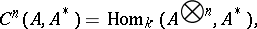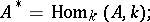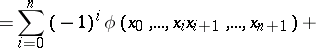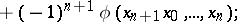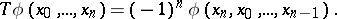The complexcomputes the Hochschild cohomology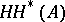ofwith values in the dual-bimodule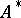. The subspace of cyclic cochains,is closed under the Hochschild coboundary mappingand the cohomology of the induced complex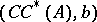is called the cyclic cohomology of the algebra, denoted by. This is a contravariant functor from the category of associative algebras to the category of linear spaces. The inclusion of complexes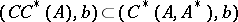gives a long exact sequence of cohomology which, in this case, has the form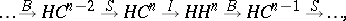and is usually called the Connes–Gysin exact sequence. The induced spectral sequence is one of the main tools for the computation of cyclic cohomology. The periodicity operatorgives rise to the stabilized version (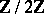-graded) of cyclic cohomology given by the direct limit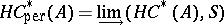and called periodic cyclic cohomology. Both cyclic and periodic cyclic cohomologies admit external products: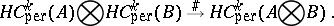Some examples are: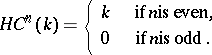Any tracial functional(i.e.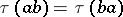for all) on the algebradefines an element of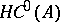.

Letbe a smooth, closed manifold and. Restricting to the continuous (in the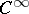-topology) cochains, one finds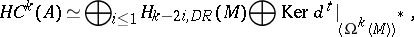whereis the space of de Rham-currents onand the operatorcoincides with the transpose of the de Rham differential. In particular, the periodic cyclic cohomology ofcoincides with de Rham homology of. An explicit mapping is given by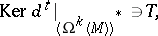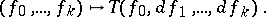The definitions given above can be easily extended to non-unital algebras.

## Properties of periodic cyclic cohomology.

Stability. The mappingis an isomorphism. This holds also for (non-periodic)) cyclic cohomology. Here,is the mapping induced by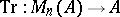from the algebra of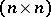-matrices overto.

Excision. Given an exact sequence of algebras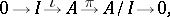there exists an associated six-term exact sequence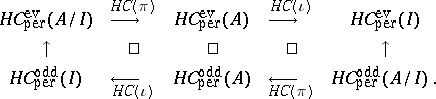The excision holds also in the case of Fréchet algebras (cf. also Fréchet algebra) if the quotient mappinghas a continuous (linear) lifting. For cyclic cohomology, under a certain condition on the ideal(called-unitality) there exists a corresponding long exact sequence.

Homotopy invariance. Suppose that, in the context of topological algebras and continuous cochains, a differentiable family of homomorphisms is given:The corresponding mappingson the periodic cyclic cohomology are-independent. In the context of cyclic cohomology the corresponding result says that inner derivations act trivially.

Pairing with-theory. Suppose thatis a Banach algebra and thatis a dense subalgebra closed under holomorphic functional calculus in. Suppose thathas been given a Fréchet topology under which its imbedding intois continuous. Restricting to continuous cochains, there exists a pairing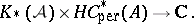On the level of idempotents, this pairing is given by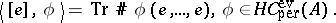This pairing is consistent with the six-term exact sequences in periodic cyclic cohomology and-theory.

Bott periodicity. In the topological context, let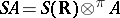be the projective tensor product ofwith the algebra of rapidly decreasing functions on. Letdenote the cyclic cocycle on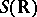given by the currentThe mapping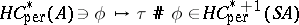is an isomorphism.

How to Cite This Entry:
Cyclic cohomology. Encyclopedia of Mathematics. URL: http://encyclopediaofmath.org/index.php?title=Cyclic_cohomology&oldid=11855
This article was adapted from an original article by R. Nest (originator), which appeared in Encyclopedia of Mathematics - ISBN 1402006098. See original article